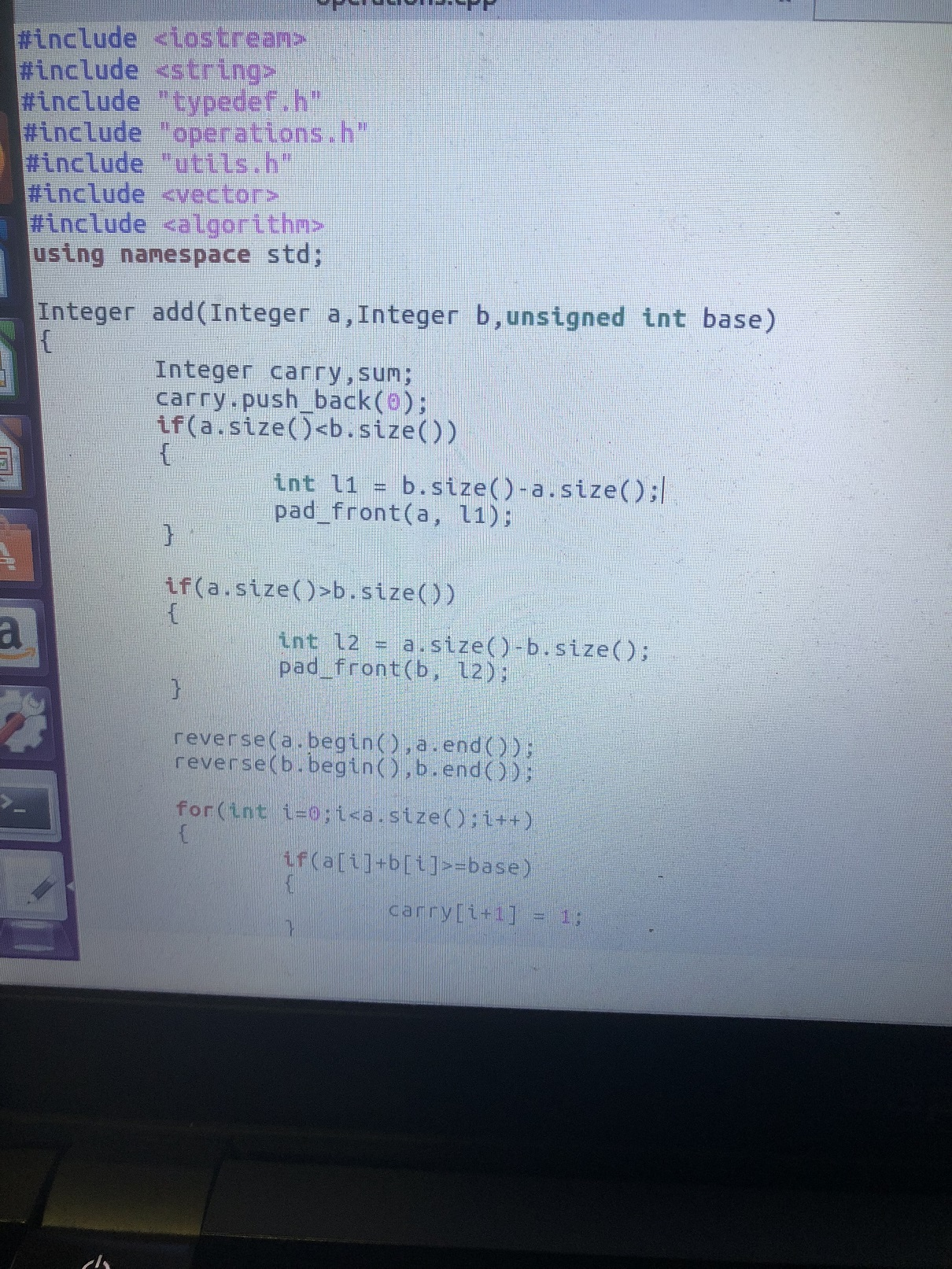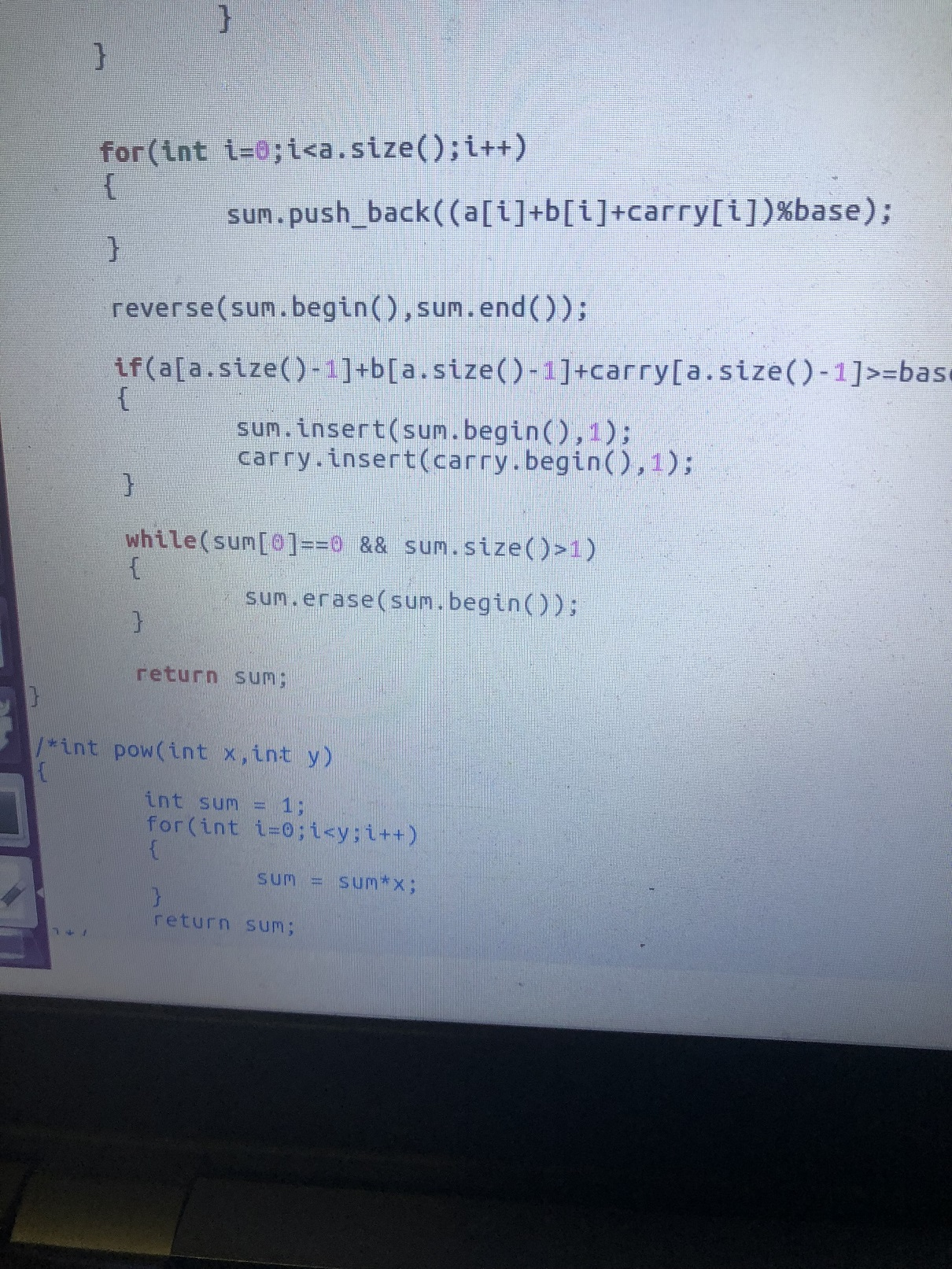c++ 运行发生malloc(): memory corruption(fast)![4个回答

`````` for(int i=0;i<a.size();i++)
{
if(a[i]+b[i]>=base)
{
//carry[i+1] = 1;
carry.push_back(1);
}
else
{
//carry[i+1] = 0;
carry.push_back(0);
}
}
``````

`````` Integer add(Integer a,Integer b,unsigned int base)
{
int len = std::max(a.size(), b.size());
Integer sum = zeros_with_digits(len + 1);

if (a.size() < b.size())
{
}

if (a.size() > b.size())
{
}

for (int i = len - 1; i >= 0; --i)
{
Digit sd = sum[i + 1] + a[i] + b[i];

sum[i + 1] = sd % base;
sum[i] = sd / base;
}

while(sum == 0 && sum.size() > 1)
{
sum.erase(sum.begin());
}

return sum;
}
``````Desol 回复caozhy: 链接: https://pan.baidu.com/s/1kZ41avRCVxnwN8P7bocFNg密码: evgjqq_41939299 是不是溢出了，超过65536，而Integer只能支持16位。Desol Integer是老师给定义的一个vector直接让我们用的，上交之后老师测试代码的数都是大于65536的，应该不是这个问题吧.

Integer add(Integer a,Integer b,unsigned int base)
{
Integer carry,sum;
carry.reverse(20);//给carry预留空间，否则后面carry[i]将访问到不存在的索引。c++ 运行发生malloc(): memory corruption(fast)

malloc(): memory corruption (fast): C++ opencv编写出错

glibc detected检测到段错误

*** glibc detected *** boa.bin: malloc(): memory corruption: 0x0048b960 *** ======= Backtrace: ========= /lib/libc.so.6[0x401ebf3c] /lib/libc.so.6[0x401eec68] /lib/libc.so.6(__libc_malloc+0xa8)[0x401f0c4c] boa.bin[0x3a2020] boa.bin[0x3a22cc] boa.bin[0x3cea74] boa.bin[0x382870] boa.bin[0x12374] boa.bin[0x13034] boa.bin[0xd1d8] /lib/libc.so.6(__libc_start_main+0x120)[0x40197fd4]

tomcat8宕掉，java8，linux系统，后台日志输出

java: malloc.c:3583: __libc_malloc: Assertion `!victim || ((((mchunkptr)((char*)(victim) - 2*(sizeof(size_t)))))->size & 0x2) || ar_ptr == (((((mchunkptr)((char*)(victim) - 2*(sizeof(size_t)))))->size & 0x4) ? ((heap_info *)((unsigned long)(((mchunkptr)((char*)(victim) - 2*(sizeof(size_t))))) & ~((2 * (4 * 1024 * 1024 * sizeof(long)))-1)))->ar_ptr : &main_arena)' failed. -bash: !victim: event not found

BOOL CImgProcess::HoughCir(int *a0,int *b0,int *r0) { int hei=GetHeight(),wid=GetWidthPixel(); int i=0,j=0,a=0,b=0,r=0,r1=0,t=0,bt=0; int Add; memset(Add,0,sizeof(int)*216000); for(i=0;i<60;i++){ for(j=0;j<60;j++){ for(a=0;a<60;a++){ Add[i][j][a]=0; } } } for(a=210;a<270;a++){ for(b=290;b<350;b++){ for(i=0;i<hei;i++){ for(j=0;j<wid;j++){ bt=GetGray(j,i); if(bt==255){ r1=(i-a)*(i-a)+(j-b)*(j-b); r=sqrt((double)r1); if(r>160&&r<220){ Add[a-210][b-290][r-160]++; } } } } } } for(a=210;a<270;a++){ for(b=290;b<350;b++){ for(r=160;r<220;r++){ if(Add[a-210][b-290][r-160]>t){ t=Add[a-210][b-290][r-160]; *a0=a; *b0=b; *r0=r; } } } } delete []Add; return TRUE; }

c语言指针及malloc申请内存问题

int** generate(int numRows, int** columnSizes, int* returnSize) { //if(numRows==0) int **result=NULL; for(int i=0;i<numRows;i++) { result[i]=(int *)malloc(sizeof(int)*i); for(int j=1;j<i;j++) result[i][j]=result[i-1][j-1]+result[i-1][j]; } return result; } 使用vs2010已经编译的时候，会在result[i]=(int *)malloc(sizeof(int)*i);这句话出错，求问一下： 1.如何对二维数组中的一位数组申请空间。 2.如果不初始化result为NULL会提示错误，原因只是空指针吗？

C语言malloc内存分配的问题

C语言内存分配malloc导致的程序退出

char *p; while (1) { p = malloc(1); *p = 0; } 这样写最后是因为没有内存退出还是向0写入退出？怎么感觉是内存完了

C语言用malloc有数量限制吗？

``` #include<stdio.h> #include<stdlib.h> #pragma warning(disable:4996) #include<time.h> //1.定义一个结构体 struct dicts { char * word; //存放单词 char * trans; //存放单词的意思 }; int main(void) { int MAXNUM = 0; char arr = { 0 }; int i = 0; struct dicts * dic; struct dicts * dic1; dic = (struct dicts *) malloc(sizeof(struct dicts)*MAXNUM); dic1 = (struct dicts *) malloc(sizeof(struct dicts)); //打开一个单词文件 FILE * fp = fopen("D:\\dict.txt", "r"); if (!fp) { printf("文件打开失败！"); return -1; } //判断dict.txt中有多少个单词 while (!feof(fp)) { fgets(arr, 1024, fp); i++; } MAXNUM = i / 2; printf("%d\n", MAXNUM); //将文件光标从新定位到开头 rewind(fp); //将所有的单词都读取到dic结构体中 i = 0; while (!feof(fp)) { printf("%d\n", i); //将单词放到dic[i].word中 memset(arr, 0, 1024); fgets(arr, 1024, fp); dic[i].word = (char *)malloc(strlen(arr) + 1); memset(dic[i].word, 0, strlen(arr) + 1); strncpy(dic[i].word, arr, strlen(arr) + 1); //将单词的意思放到dic[i].trans中 memset(arr, 0, 1024); fgets(arr, 1024, fp); dic[i].trans = (char *)malloc(strlen(arr) + 1); memset(dic[i].trans, 0, strlen(arr) + 1); strncpy(dic[i].trans, arr, strlen(arr) + 1); i++; } ........（下面代码没写，从上面的循环崩的。） ``` 为啥会崩感觉没错啊！内存也充足。就是单词个数有11万个，但是每次运行在不同的地方崩掉，有时候循环100个单词，有时候循环2000个单词。 以下是dict.txt中一部分内容： ``` #a Trans:art. 一;字母A #a.m. Trans:n. 上午 #a/c Trans:n. 往来帐户@往来:come - and - go; contact; #aardvark Trans:n. 土猪 #aardwolf Trans:n. 土狼 #aasvogel Trans:n. 秃鹰之一种 #abaci Trans:n. 算盘 #aback Trans:ad. 向后地;朝后地 #abacus Trans:n. 算盘 #abaft Trans:ad. 向船尾@prep. 在...后 #abalone Trans:n. 鲍鱼 #abandon Trans:vt. 放弃;沉溺@n. 放任 #abandoned Trans:a. 被抛弃的;自弃的;自甘堕落的 #abandonee Trans:n. 被遗弃者;被委付者 #abandoner Trans:n. 遗弃者;委付者 #abandonment Trans:n. 放弃;自暴自弃;放纵 #abas Trans:vt. 打倒 #abase Trans:vt. 降低...的地位;降低...的品格;贬抑 #abasement Trans:n. 贬抑;屈辱;谦卑 ```

c语言中，在子函数中用malloc函数开辟的空间在主函数中还有效吗?

c语言的malloc语句中动态获取的最大空间和电脑的什么有关？ c语言的malloc语句中动态获取的最大空间和电脑的什么有关？

vs2013编译c语言程序, malloc在debug下没问题, 在release模式下崩溃

RT, 还有就是如果把release版本的程序改为兼容win7模式运行的话就不崩溃正常运行, 有遇到过这样问题的吗

C语言中malloc动态分配空间的使用

c语言在子函数中malloc后想要将malloc的数组输出，该怎么做？

c语言在子函数中malloc后想要将malloc的数组输出，该怎么做？ 因为malloc我在子函数中就要释放，但是只有通过子函数我才能计算出malloc数组的大小，该怎么做？

C语言中malloc和free的问题

int *intPointer = (int *)malloc(3 * sizeof(int)); intPointer = 1; intPointer = 2; intPointer = 3; printf("before free :%ld\n",intPointer); free(intPointer); printf("after free :%ld\n",intPointer); printf("%d\t%d\t%d\n",intPointer,intPointer,intPointer); 输出结果： before free :4296037472 after free :4296037472 1 2 3 疑问：free(intPointer)之后，为什么还能通过intPointer访问malloc分配的那片内存？

C语言malloc后使用sizeof的问题

String s = new String(" a ") 到底产生几个对象？

Linux面试题（2020最新版）

JVM内存结构和Java内存模型别再傻傻分不清了

loonggg读完需要3分钟速读仅需 1 分钟大家好，我是你们的校长。我之前讲过，这年头，只要肯动脑，肯行动，程序员凭借自己的技术，赚钱的方式还是有很多种的。仅仅靠在公司出卖自己的劳动时...

85后蒋凡：28岁实现财务自由、34岁成为阿里万亿电商帝国双掌门，他的人生底层逻辑是什么？...

MySQL数据库面试题（2020最新版）

HashMap底层实现原理，红黑树，B+树，B树的结构原理 Spring的AOP和IOC是什么？它们常见的使用场景有哪些？Spring事务，事务的属性，传播行为，数据库隔离级别 Spring和SpringMVC，MyBatis以及SpringBoot的注解分别有哪些？SpringMVC的工作原理，SpringBoot框架的优点，MyBatis框架的优点 SpringCould组件有哪些，他们...